# QUESTION 10 Two conducting parallel plates have an electric field between them of magnitude 150 V/m,...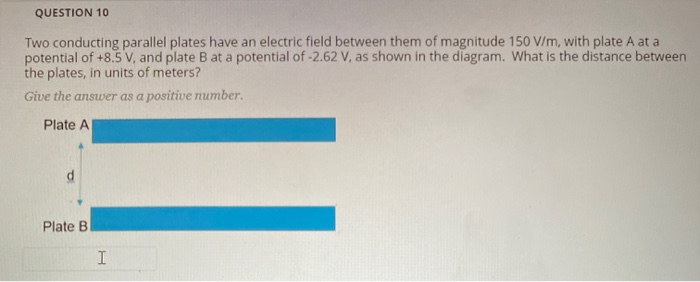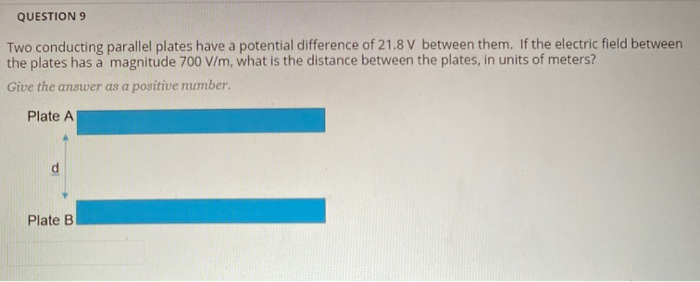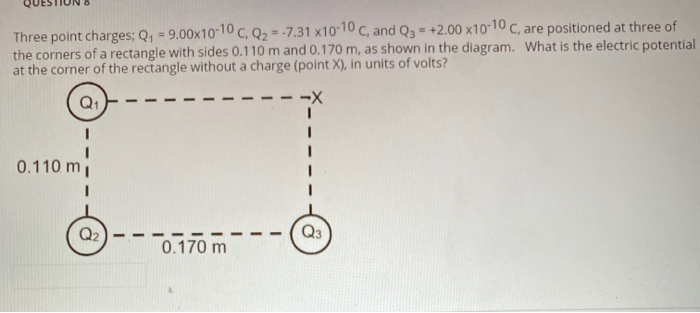QUESTION 10 Two conducting parallel plates have an electric field between them of magnitude 150 V/m, with plate A at a potential of +8.5 V, and plate B at a potential of -2.62 V, as shown in the diagram. What is the distance between the plates, in units of meters? Give the answer as a positive number. Plate A Plate B I
QUESTION 9 Two conducting parallel plates have a potential difference of 21.8 V between them. If the electric field between the plates has a magnitude 700 V/m, what is the distance between the plates, in units of meters? Give the answer as a positive number. Plate A Plate B
Three point charges; Q, = 9.00x10-10 CQ2 -.7.31 x10-10 C, and Q3 = +2.00 x10-10 C, are positioned at three of the corners of a rectangle with sides 0.110 m and 0.170 m, as shown in the diagram. What is the electric potential at the corner of the rectangle without a charge (point X), in units of volts? Q1 0.110 m Q2 0.170 m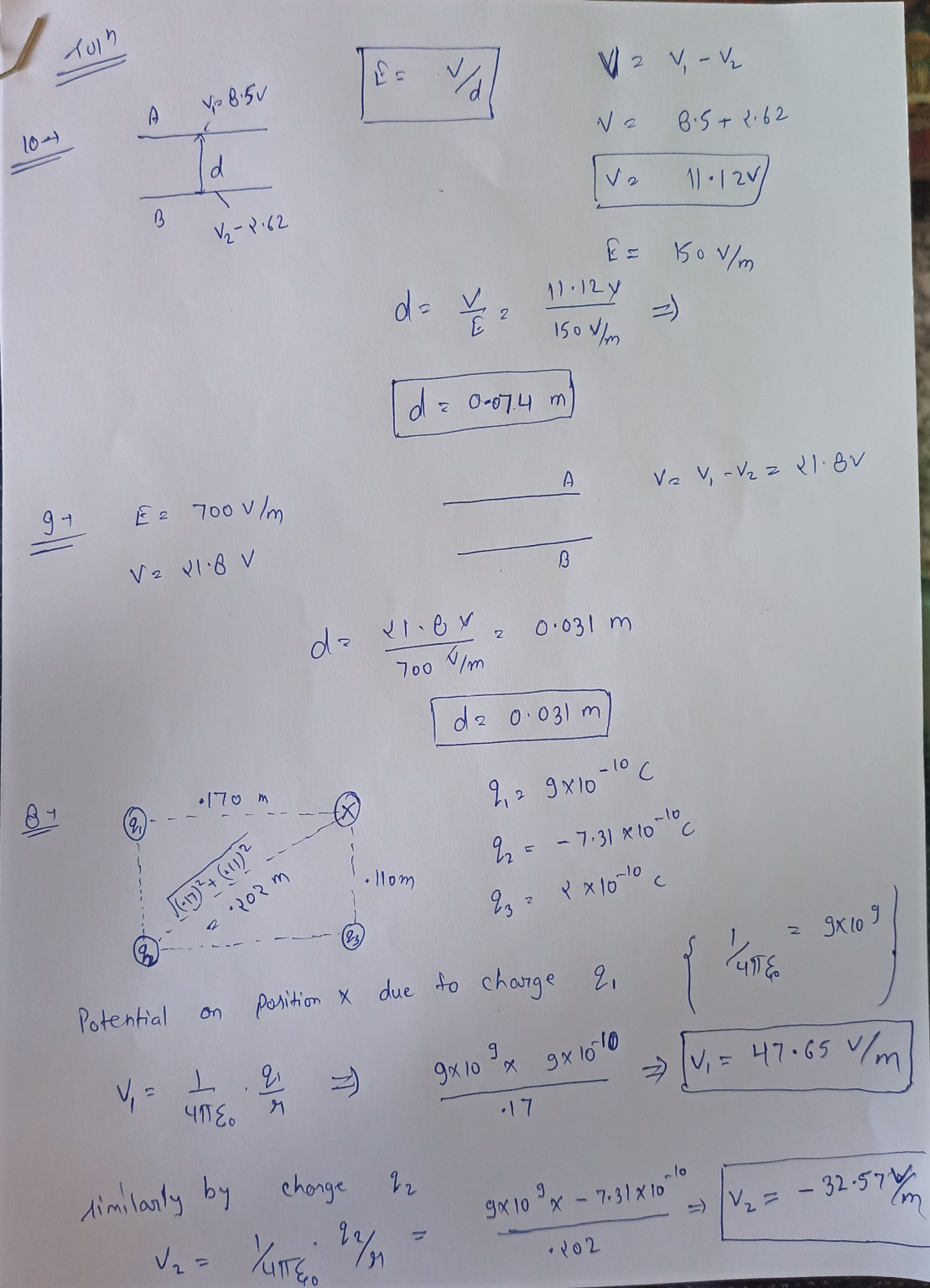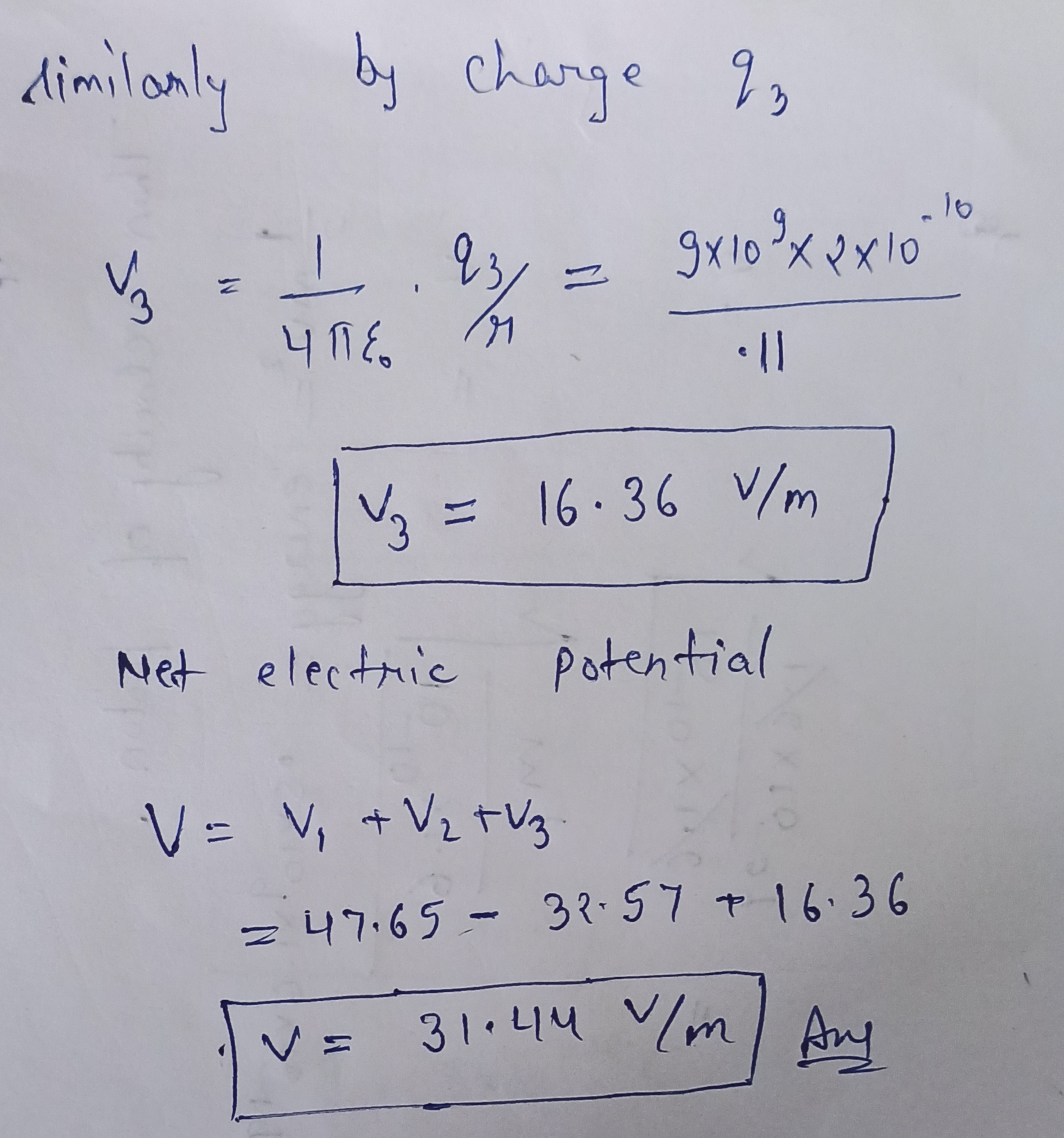#### Earn Coin

Coins can be redeemed for fabulous gifts.

Similar Homework Help Questions
• ### Two parallel conducting plates are connected to a constant voltage source. The magntude of the electric...

Two parallel conducting plates are connected to a constant voltage source. The magntude of the electric field between the plates is 2506 N/C. If the voltage is doubled and the distance between the plates is reduced to 1 4 the original distance, what is the magnitude of the new electric field? Answer in units of N/C. A pair of oppositely charged parallel plates is separated by 5.36 mm. A potential difference of 582 V exists between the plates. What is...

• ### The potential difference between two parallel conducting plates in vacuum is 350 V. An alpha particle...

The potential difference between two parallel conducting plates in vacuum is 350 V. An alpha particle with mass of 6.50 x10-27 kg and charge of 3.20 x10-19 C is released from rest near the positive plate. What is the kinetic energy of the alpha particle when it reaches the other plate? The distance between the plates is 27.0 cm.

• ### Please complete both The electric field strength between two parallel conducting plates separated by 4.20 cm...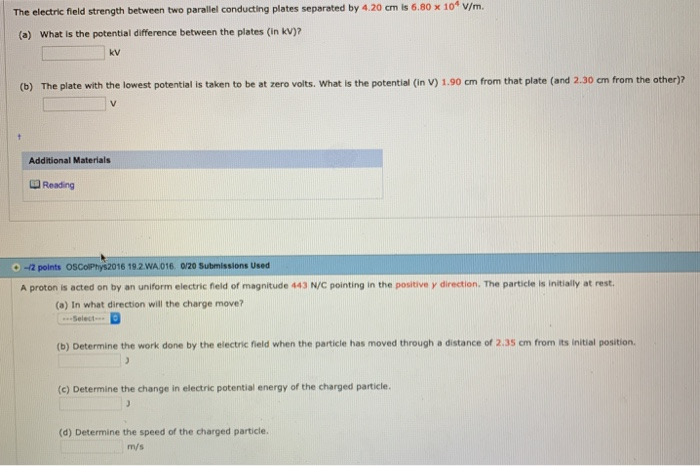Please complete both The electric field strength between two parallel conducting plates separated by 4.20 cm is 6.80 x 104 v/m. (a) What is the potential difference between the plates (in kV)2 kv (b) The plate with the lowest potential is taken to be at zero volts. What is the potential (in V) 1.90 cm from that plate (and 2.30 cm from the other)? Additional Materials Reading .-2 points oscoPhys2016 19.2.WA016 0/20 Submissions Used A proton is acted on by...

• ### What is the strength (in V/m) of the electric field between two parallel conducting plates separated...

What is the strength (in V/m) of the electric field between two parallel conducting plates separated by 1.30 cm and having a potential difference (voltage) between them of 1.45 ✕ 104 V?

• ### The electric field between two parallel plates connected to a 45‐V battery is 1300 V/m. a....

The electric field between two parallel plates connected to a 45‐V battery is 1300 V/m. a. How far apart are the plates? b. An alpha particle (which is a helium nucleus, Q =+2e,  m = 6.64 x 10‐27 kg) is released from rest at the positive plate and travels to the negative plate.    i. What is the change in potential energy of the alpha particle as it travels from the positive to the negative plate? ii. What is the speed of...

• ### Electric Potential: Two facing surfaces of two large parallel conducting plates separated...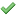Two facing surfaces of two large parallel conducting plates separated by 9.0 cm have uniform surface charge densities such thatare equal in magnitude but opposite in sign. The difference in potential between the plates is 530 V.(a) Is the positive or the negative plate at the higher potential?the positive platethe negative plate(b) What is the magnitude of the electric field between the plates?_________________ kV/m(c) An electron is released from rest next to the negatively charged surface. Find the work done...

• ### The electric potential V in the space between two flat parallel plates 1 and 2 is...

The electric potential V in the space between two flat parallel plates 1 and 2 is given (in volts) by V = 2200x2, where x (in meters) is the perpendicular distance from plate 1. At x = 1.7 cm, (a) what is the magnitude of the electric field and (b) is the field directed toward or away from plate 1?

• ### The potential difference between the two parallel plates shown in the drawing is 200 V. The...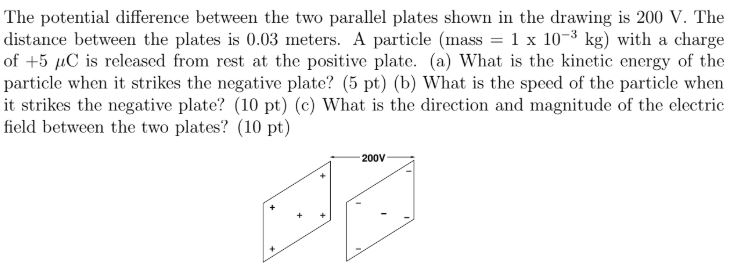The potential difference between the two parallel plates shown in the drawing is 200 V. The distance between the plates is 0.03 meters. A particle (mass = 1 x 10-3 kg) with a charge of +5 C is released from rest at the positive plate. (a) What is the kinetic energy of the particle when it strikes the negative plate? (5 pt) (b) What is the speed of the particle when it strikes the negative plate? (10 pt) (e) What...

• ### What is the strength of the electric field at the midpoint between two parallel conducting plates...

What is the strength of the electric field at the midpoint between two parallel conducting plates separated by a distance of 4.00 cm and maintained at a potential difference of 100 V?